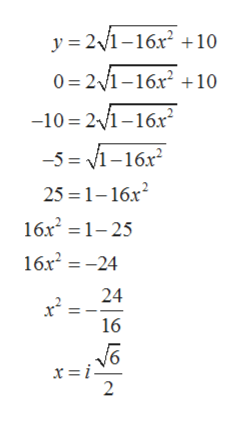# find the x and y intercepts of:f (x) = 2 (square root of 1 - 16x^2) +10

Question
30 views

find the x and y intercepts of:

(x) = 2 (square root of 1 - 16x^2) +10

check_circle

star
star
star
star
star
1 Rating
Step 1

To find the x and y intercept of the below function:

Step 2

y-intercept is a point on y when the function crosses the y-axis then the corresponding x-coordinate will be 0. Therefore, to get y-intercept substituting the value of x=0 in the given equation.
It gives:

Step 3

x-intercept is a point on x when the function crosses the X-axis then the corresponding y-coordinate will be 0. Therefore, to get x-intercept substitu...help_outlineImage Transcriptionclosey 2116x210 0 2/1-16x2 10 -10 21-16x -5 1-16x 25 1-16x 16x2 1-25 16x224 24 16 x = i- 2 fullscreen

### Want to see the full answer?

See Solution

#### Want to see this answer and more?

Solutions are written by subject experts who are available 24/7. Questions are typically answered within 1 hour.*

See Solution
*Response times may vary by subject and question.
Tagged in

### Functions